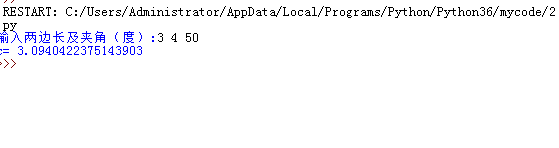﻿ python求解三角形第三边长实例_python_脚本之家
python# python求解三角形第三边长实例

## 求解三角形第三边长

```import math
x=input('输入两边长及夹角（度）:')#输入时候使用空格分隔3个值
a,b,theta=map(float,x.split())#序列解包，split()方法用于切分字符串
c=math.sqrt(a**2+b**2-2*a*b*math.cos(theta*math.pi/180))
#cos()参数为弧度
print('c=',c)
```## python三角函数用法

python中的三角函数一般有：

sin(x)返回x弧度的正弦值
cos(x)返回x弧度的余弦值
tan(x)返回x弧度的正切值
asin(x)返回x的反正弦弧度值
acos(x)返回x的反余弦弧度值
atan(x)返回x的反正切弧度值

360°=2π弧度，因此，1弧度约为57.3°，即57°17’44.806"。

• 1° = π/180弧度
• 180° = π弧度

（在python中， pi = π，它是数学常量，引用时不要忘了math!）

```import math
a = math.cos(math.pi)    #求cos(180°)
b = math.sin(math.pi/2)  #求sin(90°）
print(a,b)
"""

-1.0  1.0
"""```

• `degrees(x)` : 将弧度转化为角度。
• `radians(x)` : 将角度转化为弧度。

```import math
a = math.degrees(math.pi/2)
print(a)